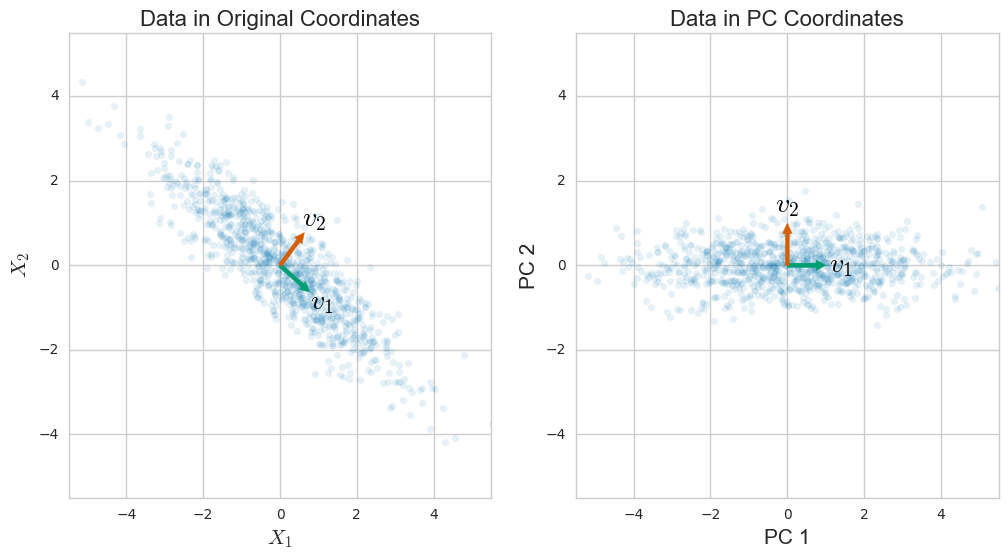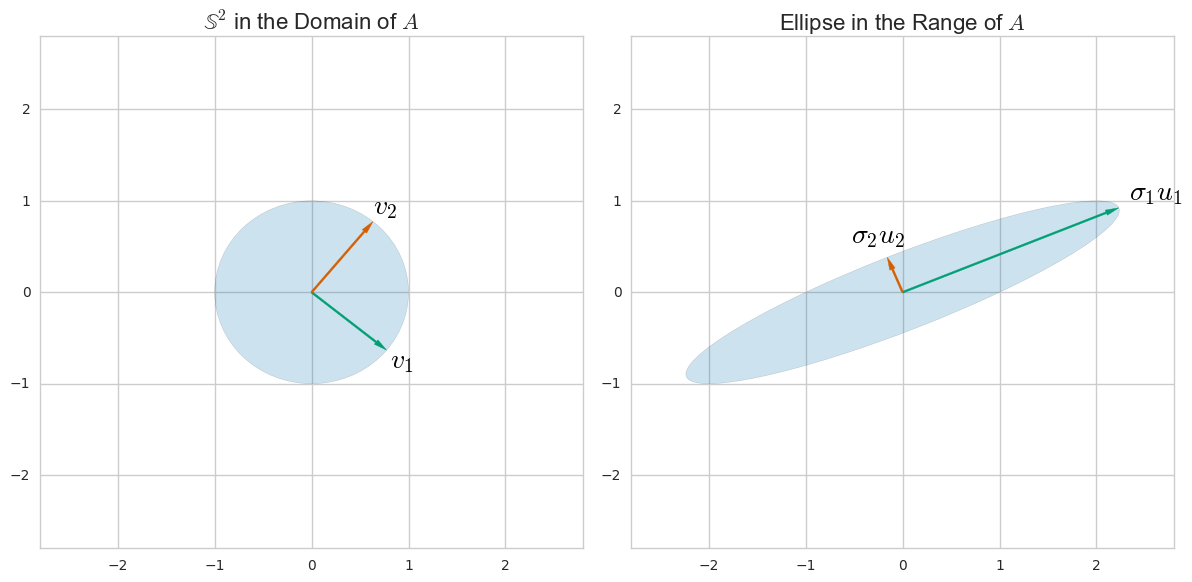# SVD与PCA之间的关系。如何使用SVD执行PCA？

351

8

2

ttnphns

PCA是SVD的特例。PCA需要标准化的数据，最好是同一单位。在PCA中，矩阵为nxn。
Orvar Korvar

@OrvarKorvar：您在说什么nxn矩阵？
Cbhihe

411

$\mathbf{C}=\mathbf{V}\mathbf{L}{\mathbf{V}}^{\mathrm{\top }},$
$\mathbf{V}$$\mathbf V$$\mathbf{L}$$\mathbf L$${\lambda }_{i}$$\lambda_i$; 这些可以看作是新的，转换后的变量。第个主成分由第列给出。新的PC空间中第个数据点的坐标由第行给出。$j$$j$$j$$j$$\mathbf{X}\mathbf{V}$$\mathbf {XV}$$i$$i$$i$$i$$\mathbf{X}\mathbf{V}$$\mathbf{XV}$

$\mathbf{X}=\mathbf{U}\mathbf{S}{\mathbf{V}}^{\mathrm{\top }},$
$\mathbf{U}$$\mathbf U$$\mathbf{S}$$\mathbf S$${s}_{i}$$s_i$
$\mathbf{C}=\mathbf{V}\mathbf{S}{\mathbf{U}}^{\mathrm{\top }}\mathbf{U}\mathbf{S}{\mathbf{V}}^{\mathrm{\top }}/\left(n-1\right)=\mathbf{V}\frac{{\mathbf{S}}^{2}}{n-1}{\mathbf{V}}^{\mathrm{\top }},$
$\mathbf{V}$$\mathbf V$${\lambda }_{i}={s}_{i}^{2}/\left(n-1\right)$$\lambda_i = s_i^2/(n-1)$$\mathbf{X}\mathbf{V}=\mathbf{U}\mathbf{S}{\mathbf{V}}^{\mathrm{\top }}\mathbf{V}=\mathbf{U}\mathbf{S}$$\mathbf X \mathbf V = \mathbf U \mathbf S \mathbf V^\top \mathbf V = \mathbf U \mathbf S$

1. 如果，则主要方向/轴。$\mathbf{X}=\mathbf{U}\mathbf{S}{\mathbf{V}}^{\mathrm{\top }}$$\mathbf X = \mathbf U \mathbf S \mathbf V^\top$$\mathbf{V}$$\mathbf V$
2. 列是主要组成部分（“分数”）。$\mathbf{U}\mathbf{S}$$\mathbf {US}$
3. 奇异值通过与协方差矩阵的特征值相关。特征值显示各个PC的方差。${\lambda }_{i}={s}_{i}^{2}/\left(n-1\right)$$\lambda_i = s_i^2/(n-1)$${\lambda }_{i}$$\lambda_i$
4. 标准化分数由列给出，负载由。例如，请参见此处此处，了解为什么不应将“负载”与主要说明混淆。$\sqrt{n-1}\mathbf{U}$$\sqrt{n-1}\mathbf U$$\mathbf{V}\mathbf{S}/\sqrt{n-1}$$\mathbf V \mathbf S/\sqrt{n-1}$
5. 仅当居中时，以上才是正确的。$\mathbf{X}$$\mathbf X$只有这样，协方差矩阵才等于。${\mathbf{X}}^{\mathrm{\top }}\mathbf{X}/\left(n-1\right)$$\mathbf X^\top \mathbf X/(n-1)$
6. 以上仅适用于在行中具有样本而在列中具有变量的情况。如果变量在行中，样本在列中，则和交换解释。$\mathbf{X}$$\mathbf X$$\mathbf{U}$$\mathbf U$$\mathbf{V}$$\mathbf V$
7. 如果要在相关矩阵（而不是协方差矩阵）上执行PCA，则列不仅应居中，而且也应标准化，即除以它们的标准偏差。$\mathbf{X}$$\mathbf X$
8. 要将数据的维数从减小到，请选择前列，并选择的左上部分。他们的乘积是包含前 PC的矩阵。$p$$p$$k$k$k$$k$$\mathbf{U}$$\mathbf U$$k×k$$k\times k$$\mathbf{S}$$\mathbf S$${\mathbf{U}}_{k}{\mathbf{S}}_{k}$$\mathbf U_k \mathbf S_k$$n×k$$n \times k$$k$$k$
9. 将前 PC 乘以相应的主轴得出矩阵具有原始 x 大小，但等级较低（等级）。该矩阵提供了前 PC 的原始数据的重构。它具有尽可能低的重建误差，请在此处查看我的答案$k$$k$${\mathbf{V}}_{k}^{\mathrm{\top }}$$\mathbf V_k^\top$${\mathbf{X}}_{k}={\mathbf{U}}_{k}^{\phantom{\mathrm{\top }}}{\mathbf{S}}_{k}^{\phantom{\mathrm{\top }}}{\mathbf{V}}_{k}^{\mathrm{\top }}$$\mathbf X_k = \mathbf U_k^\vphantom \top \mathbf S_k^\vphantom \top \mathbf V_k^\top$$n×p$$n \times p$$k$$k$${\mathbf{X}}_{k}$$\mathbf X_k$$k$$k$
10. 严格来说，的大小是，大小是。但是，如果则的最后列是任意的（并且对应行为常数零）；因此应该使用的经济尺寸（或返回）SVD的的大小，丢弃所述无用列。对于较大的矩阵否则将不必要地巨大。对于的相反情况也是如此$\mathbf{U}$$\mathbf U$$n×n$$n\times n$$\mathbf{V}$$\mathbf V$$p×p$$p \times p$$n>p$$n>p$$n-p$$n-p$$\mathbf{U}$$\mathbf U$$\mathbf{S}$$\mathbf S$$\mathbf{U}$$\mathbf U$$n×p$$n\times p$$n\gg p$$n\gg p$$\mathbf{U}$$\mathbf U$$n\ll p$$n\ll p$

## 其他连结5
@Antoine，协方差矩阵的定义等于，其中尖括号表示平均值。如果将所有作为行堆叠在一个矩阵，则此表达式等于。如果居中，则简化为。考虑方差；等于。但是，如果（即数据居中），则它只是的平均值。$⟨\left({\mathbf{x}}_{i}-\overline{\mathbf{x}}\right)\left({\mathbf{x}}_{i}-\overline{\mathbf{x}}{\right)}^{\mathrm{\top }}⟩$$\langle (\mathbf x_i - \bar{\mathbf x})(\mathbf x_i - \bar{\mathbf x})^\top \rangle$${\mathbf{x}}_{i}$$\mathbf x_i$$\mathbf{X}$$\mathbf X$$\left(\mathbf{X}-\overline{\mathbf{X}}\right)\left(\mathbf{X}-\overline{\mathbf{X}}{\right)}^{\mathrm{\top }}/\left(n-1\right)$$(\mathbf X - \bar{\mathbf X})(\mathbf X - \bar{\mathbf X})^\top/(n-1)$$\mathbf{X}$$\mathbf X$$\mathbf{X}{\mathbf{X}}^{\mathrm{\top }}/\left(n-1\right)$$\mathbf X \mathbf X^\top/(n-1)$$⟨\left({x}_{i}-\overline{x}{\right)}^{2}⟩$$\langle (x_i-\bar x)^2 \rangle$$\overline{x}=0$$\bar x=0$${x}_{i}^{2}$$x_i^2$

1

ttnphns

2
@amoeba是的，但是为什么要使用它？另外，是否可以对使用相同的分母？问题是我看到公式并尝试理解，如何使用它们？$S$$S$${\lambda }_{i}={s}_{i}^{2}$$\lambda_i = s_i^2$

1
@sera只需转置矩阵并摆脱您的问题。否则，您只会感到困惑。

22

import numpy as np
from numpy import linalg as la
np.random.seed(42)

def flip_signs(A, B):
"""
utility function for resolving the sign ambiguity in SVD
http://stats.stackexchange.com/q/34396/115202
"""
signs = np.sign(A) * np.sign(B)
return A, B * signs

# Let the data matrix X be of n x p size,
# where n is the number of samples and p is the number of variables
n, p = 5, 3
X = np.random.rand(n, p)
# Let us assume that it is centered
X -= np.mean(X, axis=0)

# the p x p covariance matrix
C = np.cov(X, rowvar=False)
print "C = \n", C
# C is a symmetric matrix and so it can be diagonalized:
l, principal_axes = la.eig(C)
# sort results wrt. eigenvalues
idx = l.argsort()[::-1]
l, principal_axes = l[idx], principal_axes[:, idx]
# the eigenvalues in decreasing order
print "l = \n", l
# a matrix of eigenvectors (each column is an eigenvector)
print "V = \n", principal_axes
# projections of X on the principal axes are called principal components
principal_components = X.dot(principal_axes)
print "Y = \n", principal_components

# we now perform singular value decomposition of X
# "economy size" (or "thin") SVD
U, s, Vt = la.svd(X, full_matrices=False)
V = Vt.T
S = np.diag(s)

# 1) then columns of V are principal directions/axes.
assert np.allclose(*flip_signs(V, principal_axes))

# 2) columns of US are principal components
assert np.allclose(*flip_signs(U.dot(S), principal_components))

# 3) singular values are related to the eigenvalues of covariance matrix
assert np.allclose((s ** 2) / (n - 1), l)

# 8) dimensionality reduction
k = 2
PC_k = principal_components[:, 0:k]
US_k = U[:, 0:k].dot(S[0:k, 0:k])
assert np.allclose(*flip_signs(PC_k, US_k))

# 10) we used "economy size" (or "thin") SVD
assert U.shape == (n, p)
assert S.shape == (p, p)
assert V.shape == (p, p)

21

$X=\left(\begin{array}{ccccc}& & {x}_{1}^{T}-{\mu }^{T}& & \\ & & {x}_{2}^{T}-{\mu }^{T}& & \\ & & ⋮& & \\ & & {x}_{n}^{T}-{\mu }^{T}& & \end{array}\right)\phantom{\rule{thinmathspace}{0ex}}.$

$S=\frac{1}{n-1}\sum _{i=1}^{n}\left({x}_{i}-\mu \right)\left({x}_{i}-\mu {\right)}^{T}=\frac{1}{n-1}{X}^{T}X$

$S=V\mathrm{\Lambda }{V}^{T}=\sum _{i=1}^{r}{\lambda }_{i}{v}_{i}{v}_{i}^{T}\phantom{\rule{thinmathspace}{0ex}},$SVD是一种根据矩阵的列空间和行空间来理解矩阵的一般方法。（这是一种以与行和列空间具有直观关系的其他矩阵形式重写任何矩阵的方法。）例如，对于矩阵我们可以在域和范围内找到和方向，以便$A=\left(\begin{array}{cc}1& 2\\ 0& 1\end{array}\right)$$A = \left( \begin{array}{cc}1&2\\0&1\end{array} \right)$${u}_{i}$$u_i$${v}_{i}$$v_i$$A$$A$$\mathbb{S}$$\mathbb S$${u}_{i}$$u_i$${v}_{i}$$v_i$

$X$$X$$A=X$$A = X$

$X=\sum _{i=1}^{r}{\sigma }_{i}{u}_{i}{v}_{j}^{T}\phantom{\rule{thinmathspace}{0ex}},$

$\left\{{u}_{i}\right\}$$\{ u_i \}$$\left\{{v}_{i}\right\}$$\{ v_i \}$$S$$S$${v}_{i}$$v_i$

${u}_{i}=\frac{1}{\sqrt{\left(n-1\right){\lambda }_{i}}}X{v}_{i}\phantom{\rule{thinmathspace}{0ex}},$

${\sigma }_{i}^{2}=\left(n-1\right){\lambda }_{i}\phantom{\rule{thinmathspace}{0ex}}.$